Subitizing is the ability to recognise the total number of objects in a group without counting them.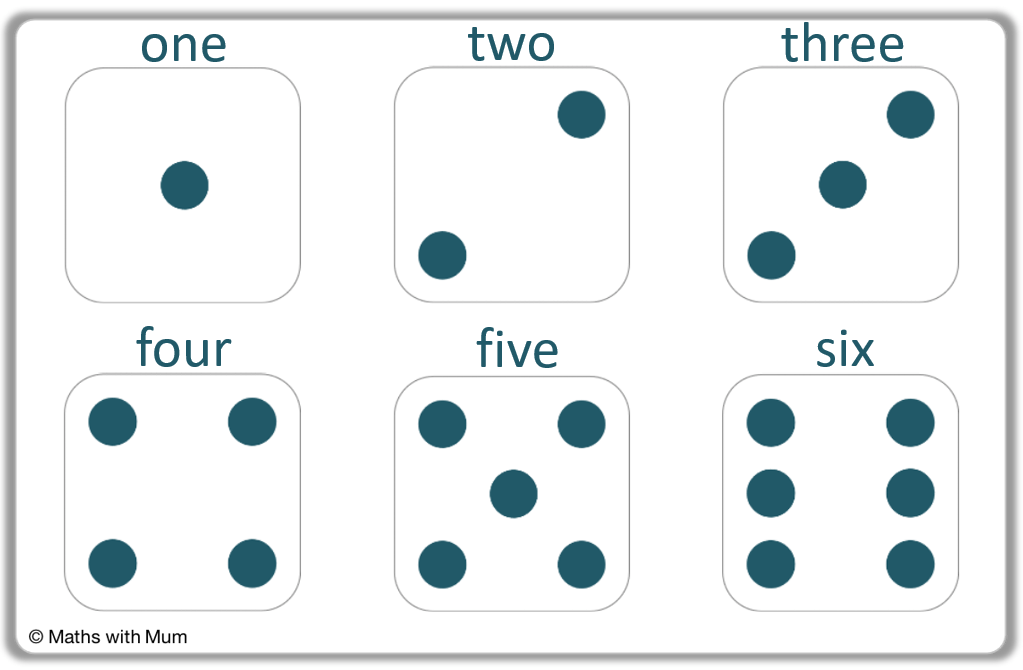• Subitizing is the ability to see a total number of objects just by looking at them and not counting them individually.
• Subitizing involves recognising the image of a number, so that the total can be seen immediately.
• Rolling a dice is an example of how subitizing is used. We recognise the pattern of dots on each face rather than counting the individual dots.
• Subitizing is important as it helps to build the skills of counting, skip counting, understanding numbers as part of groups and partitioning numbers.

Here is an example of using flashcards to subitize the number 2.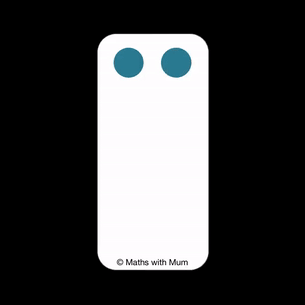#### Subitizing Flash Cards#### Subitizing to 10 Video#### Subitizing Flashcards Online

Subitizing Flashcards Online Interactive Activity

# Subitizing

## What is Subitizing?

Subitizing is the ability to recognise the total number of objects in a group without counting them. For example, when rolling a die we know the number without counting the dots on each face.

Here are the dot patterns on a standard dice.When we roll a dice, we do not usually count the dots on each face. Instead we recognise the pattern.

Being able to recognise the number without counting it is known as subitizing.

The word subitize is pronounced ‘soo-bit-ize’. It derives from the Latin word ‘subitus’, which means sudden. This is because when subitizing, we know the total ‘suddenly’. We do not need to take time to count the total.

## Why Subitizing is Important

Subitizing is an important mathematical skill where a total can be recognised without counting. It helps us to develop number sense, counting on, skip counting, grouping and partitioning. It also helps to develop pattern recognition.

Once we can subitize smaller numbers, it is possible to look for these patterns when subitizing larger numbers.

For example, to subitize 6, we would notice the two patterns of 3 and then recognise that this makes 6.Subitizing helps us to spot pairs or groups of three. This can help us to count up in twos, threes or fives more easily and it builds the skills needed for skip counting.

## How to Teach Subitizing

The following ideas will help with teaching subitizing:

• Dice games
• Domino games
• Five and ten frames
• Flashcard games
• Using manipulatives

The process of teaching subitizing involves exposing children to images of collections of objects in order to increase their familiarity with the image of each number. To improve your ability to subitize, increase your exposure to collections of numbers in different situations and forms.

#### Subitizing with Dice

Subitizing is the ability to recognise the number on a dice without counting the dots. To practise subitizing with a dice, play lots of games involving dice and saying the number. It helps to view the whole face as a pattern or image that can be remembered.

Dice games, such as snakes and ladders or Yahtzee familiarise children with subitizing in a fun way.#### Subitizing with Dominoes

Subitizing is the ability to recognise the number on a domino without counting the dots. To practise subitizing with dominoes, play lots of games involving dominoes, particularly ones that involve matching two of the same number. This helps to reinforce the image of each number.

Dominoes also display numbers in a similar arrangement to dice. Matching common numbers on two different dominoes is another way to practise subitizing.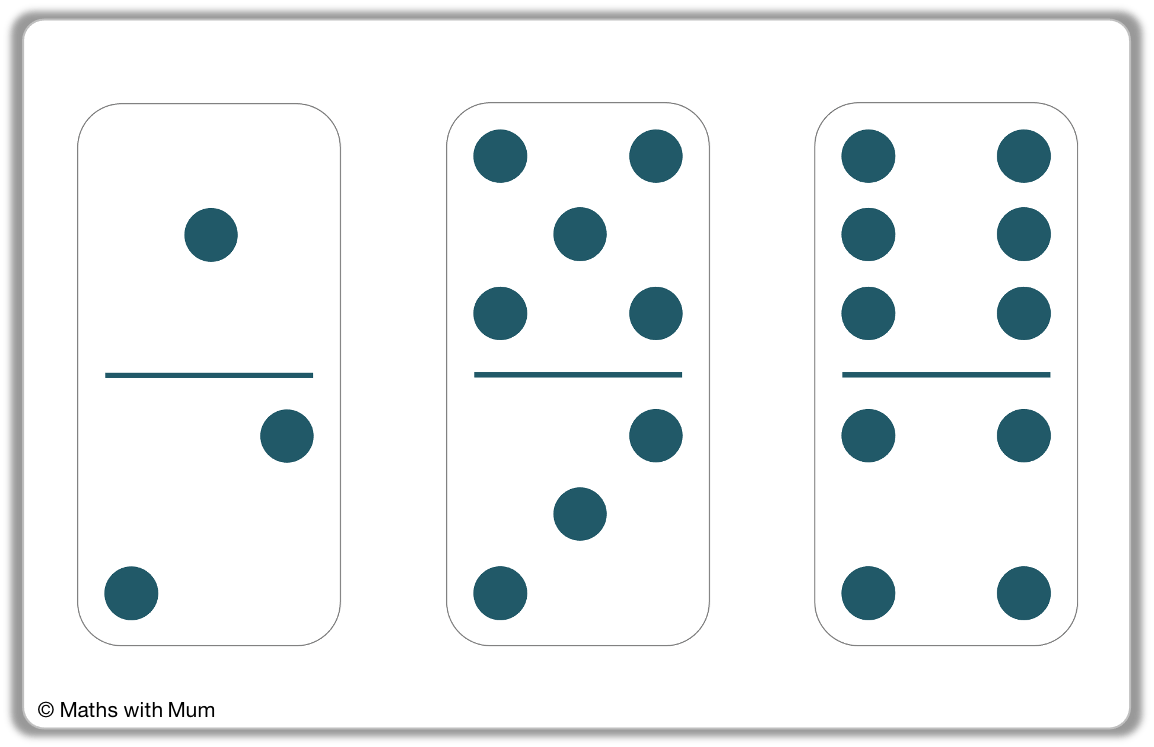#### Subitizing with Ten Frames

Ten frames are grids arranged in 2 rows of 5 squares. The familiarity of the frame helps us to better recognise the numbers being represented and hence, improve our subitizing skill. When first learning to subitize, it is useful to start with five frames which are just one row of the tens frame.Ten frames are a simple way to represent numbers. Children may find it useful to use ten frames when subitizing because the longer the number, the larger it is. This can help speed up the subitizing process. Seeing a full row of 5 may be an easier way to visualise 5 compared to a randomly allocated set of objects.

Tens frames can allow us to more easily subitize the numbers 6 to 10. Since we can subitize 1 and 2 easily, if we see 1 or 2 next to a complete row of 5, we know that we have 6 or 7.

We can subitize 10 easily on a tens frame because it is a completely full two rows.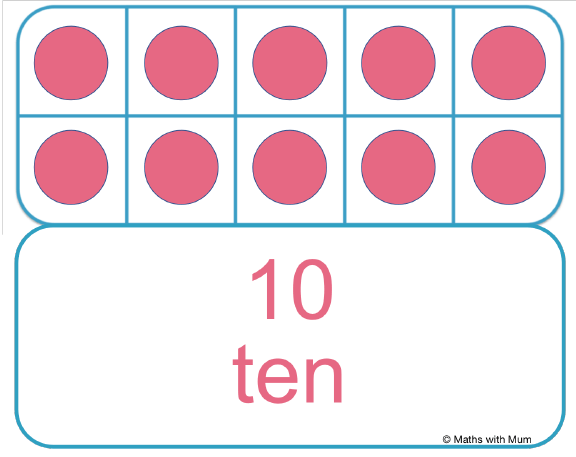We can also subitize 8 and 9 easily on a tens frame because we can subitize the missing squares in the frame. If we are missing one square, we must have 9 and if we are missing 2 squares, we must have 8.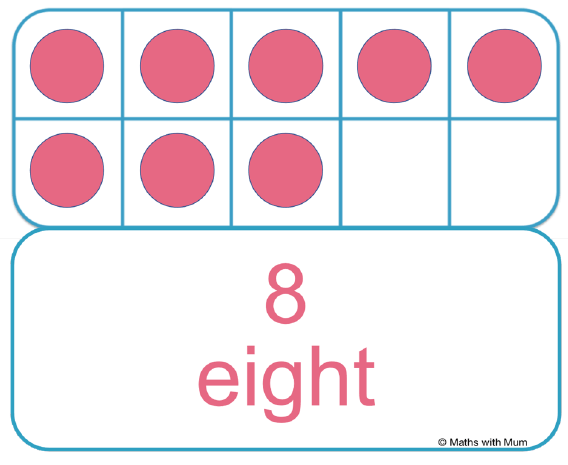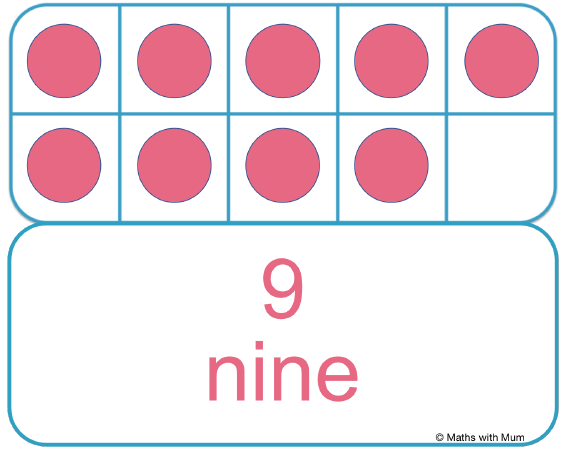#### Subitizing Flashcards

Subitizing flashcards contain an image of 1-5 objects on the front of the card and the corresponding number printed on the back. The purpose is to practise subitizing the image of the objects and then turning the cards over to see if it was correct. Subitizing cards can be used in games such as snap or memory games.

Flashcards can be the easiest way to practise subitizing. Simply print the flashcards above or use the interactive online subitizing game above.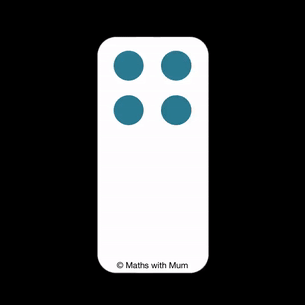#### Subitizing with Manipulatives

Manipulatives are physical objects that are used to represent amounts. Manipulatives are particularly useful for practising subitizing because children can move them around and see totals represented in a variety of different forms rather than in the same fixed pattern as seen on dice or ten frames.

Games involving manipulatives are another great way to practise subitizing. Using lego blocks, counters or balls of plasticine are great for children to use as they can move these items around and see the collection of objects in many different forms.

## Subitizing Tally Marks

Subitizing is the ability to recognise a number without counting. Tally marks are an example where subitizing may be used. For example, when we see a collection of 4 lines with a diagonal across it, we immediately know we have a tally of 5 and we do not need to count each individual line.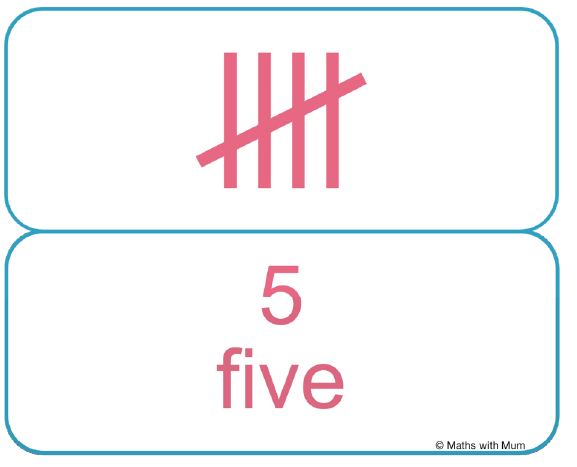As soon as we see a tally with a diagonal line striking through it, we know it represents 5. This allows us to see the collection as an image and hence, subitize. We would not count the individual lines to see that there are 5. Using tally marks encourages us to subitize.

## Subitizing Large Numbers

Many people can subitize numbers 1-5. To subitize larger numbers, visualise them as groups of the numbers 1-5. For example, 6 can be seen as two groups of 3. Then 7 can be subitized by considering it as a group of 6 with 1 more. To subitize larger numbers, recognise the combinations of smaller numbers.

#### Subitizing One

Here is a subitizing flashcard for one.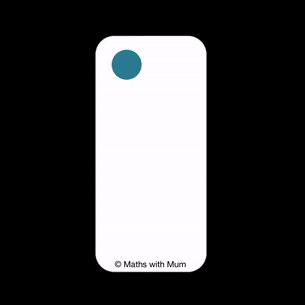#### Subitizing Two

Here is a subitizing flashcard for two.#### Subitizing Three

Here is a subitizing flashcard for three.#### Subitizing Four

Here is a subitizing flashcard for four.It may be useful to think of four as two groups of two. If you can subitize two, then look for two groups of two when subitizing four.

#### Subitizing Five

Here is a subitizing flashcard for five.The largest number that most people can subitize is 5. We can think of 5 as a group of 3 next to a group of 2, so if we can subitize to see a group of 3 and a group of 2, then we can remember that this makes 5.

Alternatively, it can be helpful to start by displaying five in same arrangement shown on a dice face. If we recognise a square of four dots with one in the middle, this can be a familiar image to use when subitizing 5.#### Subitizing Six

Here is a subitizing flashcard for six.6 may be subitized by recognising two groups of 3. It is particularly helpful to recognise two rows or columns of three if the objects are lined up alongside another in pairs.#### Subitizing Seven

Here is a subitizing flashcard for seven.To subitize 7, we can look for a group of 4 alongside a group of 3. If we can subitize 4 and 3, we can recognise that both together make 7.Alternatively, we can subitize 7 as a group of 6 with one extra.

#### Subitizing Eight

Here is a subitizing flashcard for eight.Since 4 is easily subitized, we can subitize 8 as two groups of 4. The groups of 4 as a square might be easier to use when subitizing 8.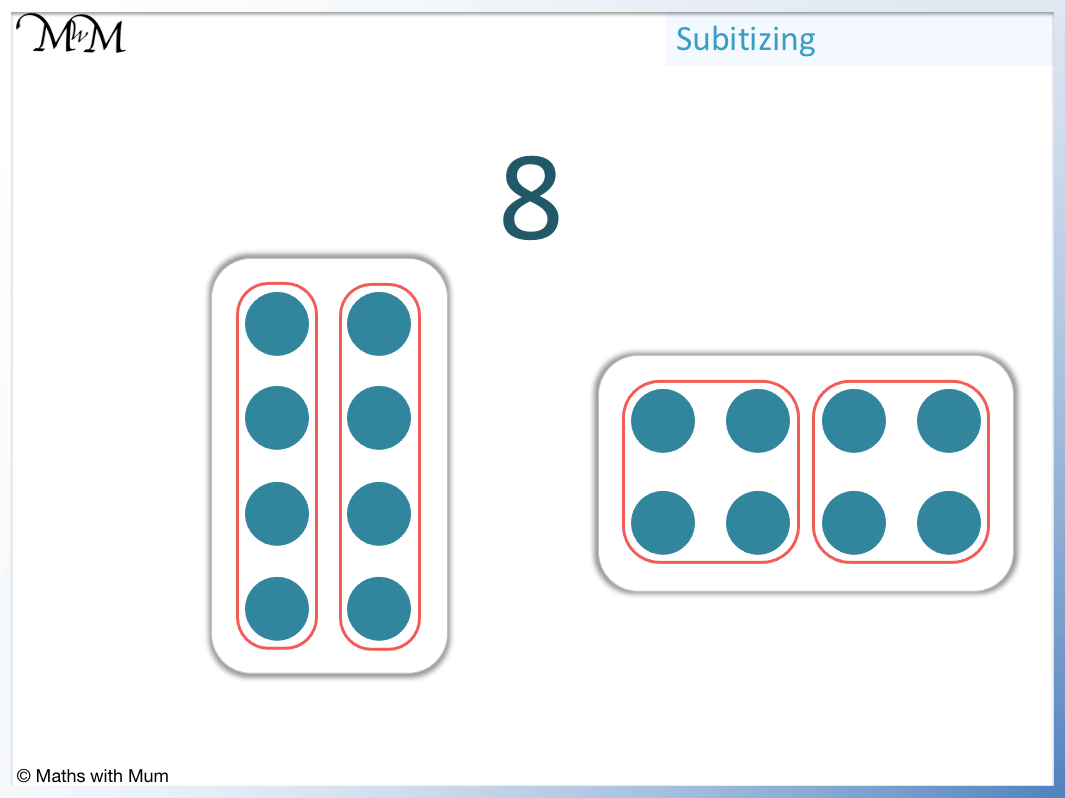Alternatively, we can subitize 8 as being 2 less than a complete tens frame. This is because 8 is 2 less than 10 and 2 is easier to subitize. If we can see 2 gaps on a tens frame, we know we have 8.#### Subitizing Nine

Here is a subitizing flashcard for nine.We can subitize nine as a group of 4 and a group of 5 together.Alternatively, we can more easily subitize 9 as one less than 10 if using a tens frame. If we see that one is missing from a complete tens frame, then we know we have 9.#### Subitizing Ten

Here is a subitizing flashcard for ten.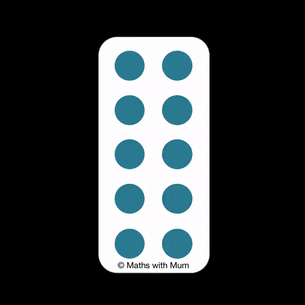Ten can be subitized by visualising it as two groups of 5. It can be easier to visualise each group of 5 as a square of four with a single dot in between.Now try our lesson on Skip Counting by 2 where we learn how to count up in twos.error: Content is protected !!